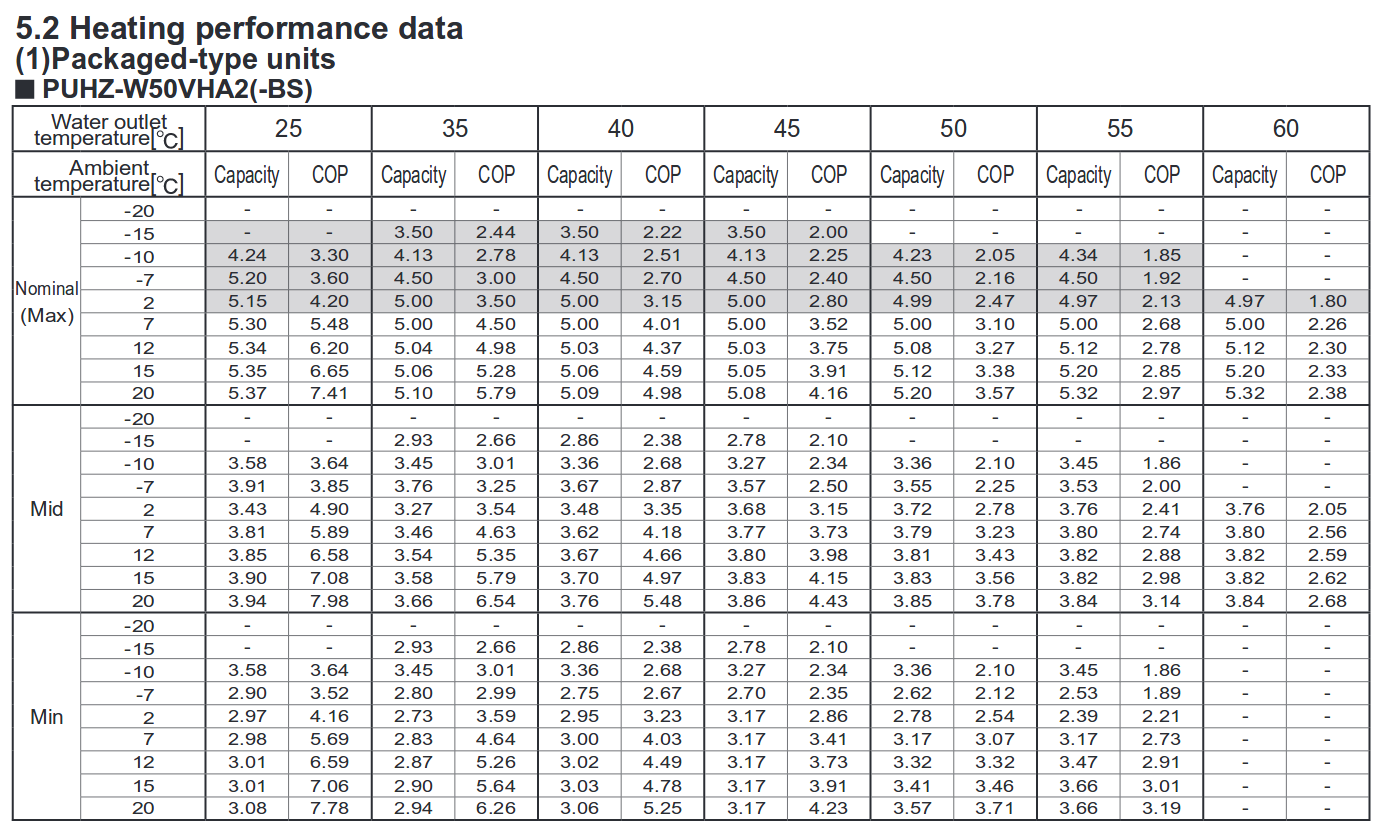Published: 4th January, 2018

Updated: 30th November 2020

Heat pumps have higher performance at low flow temperatures (see example performance table below for a 5kW EcoDan ASHP). However the heat output from radiators is greatly reduced at lower water temperatures and so to achieve an efficient system it is usually the case that the radiator area in each room needs to be increased.

When you reduce the mean water temperature of a radiator its heat output does not reduce linearly. The heat output at a Delta_T of 25K (half the standard test Delta_T of 50K) is less than half of the heat output given at 50K. The heat output given by a radiator at different values of Delta_T is usually determined using a correction factor table:

Delta_T Correction Factor
20 0.3
25 0.41
30 0.52
35 0.63
40 0.75
45 0.87
50 1

*Correction factor's from Heatpumps for the home by John Cantor taken from manufacturer's data.

To determine the correction factor at smaller increments of Delta_T the following equation gives a good fit to the above data:

Correction Factor = (Delta_T / Rated_Delta_T) ^ 1.3
Heat_output = Rated_Heat_Output x (Delta_T / Rated_Delta_T) ^ 1.3

The heat output of a radiator is determined by its mean water temperature (MWT). Which should be the average temperature across the radiator surface. Its important to make the distinction between the flow and return temperatures coming out or in to a heatpump and the mean water temperature of the radiator which will likely be somewhere in the middle of the two.

For example a heatpump producing an outlet/flow temperature of 35C combined with a heat emitter system that results in an inlet/return temperature of 30C, is likely to result in a radiator mean water temperature of (35+30)/2 = 32.5C.

Heat output from a double panel convector radiator

A double panel convector such as a standard screwfix kudox double panel, double convector 1200x600 has a rated output of 2146W (2980 W/m2), 1.74x the heat output of an equivalent dimensioned single panel convector. Applying the correction factor equation above gives the expected heat output at the lower mean water temperatures that give better heat pump performance:

MWT 30C (20C room) = 2146W x (10K / 50K) ^ 1.3 = 265W
MWT 35C (20C room) = 2146W x (10K / 50K) ^ 1.3 = 449W
MWT 40C (20C room) = 2146W x (10K / 50K) ^ 1.3 = 652W
MWT 45C (20C room) = 2146W x (10K / 50K) ^ 1.3 = 871W

As we can see, running at 30C which would give a really good COP provides 8x less heat output than at the radiator design temperature of 70C! This means that either you either need much larger radiators, more radiators or/and longer heating system run times to provide the same amount of heat energy into the house.

I've been able to make it work with these standard screwfix kudox double panel radiators in our house, see the Room by room heat loss calculation and radiator sizing on this page. If you cant make it work, its worth considering fan assisted radiators which give much greater heat outputs from much more compact radiators dimensions such as the Dimplex SmartRad.

5kW EcoDan ASHP Performance at different operating conditions

The following table provides the COP performance for a 5kW EcoDan ASHP at different flow temperatures, external temperatures and compressor speed (min, mid, max). Notice how the best performance is obtained at lower flow temperatures, higher outside temperatures and mid range compressor speed.